2019-03-04 15:13:02 qq_33612918 阅读数 146
• ###### AI时代，机器学习该如何入门？

机器学习入门视频教程，该课程会告诉大家如何入门机器学习，掌握机器学习必要的基础知识，了解机器学习路径。对于机器学习，很多人的观点是：机器学习技术是今后所有技术人员都绕不过的一个门槛。 那么，普通程序员该学习机器学作为一名对机器学习心有向往的程序员，我该以什么样的姿势开始呢？不妨看看该课程。

7185 人正在学习 去看看 CSDN讲师

## 原理

1. 先向东西方向看，下降最大的方向是哪边（这个步骤叫求 a 的偏导）
2. 再向南北方向看，下降最大的方向是哪边（这个步骤叫求 b 的偏导）
3. 向确定的方向跨 α 步 （这个 α 叫做步长）
4. 不断重复步骤123，确定四周已经足够平滑（设置 误差 ，表示已经到最低点了）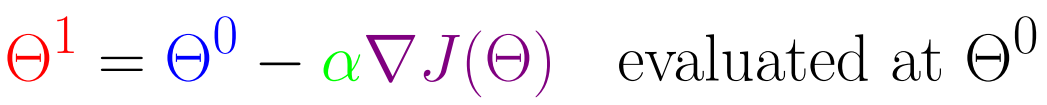## 代码实现

// 一元线性回归
// _a, _b 	—— 需求的参数值
// xi, yi 	—— 样本数组
// _step 	—— 步长设置
// _inaccuracy —— 最大误差
function gradient_descent(_a, _b, xi, yi, _step, _inaccuracy) {
let a = _a	//设置初始值
let	b = _b	//设置初始值
const step = _step					// 定义步长
const inaccuracy = _inaccuracy		// 定义最大误差
let count = 400						// 最大迭代次数，防止无限循环
while (count--){
let grad_a = Ja(a, b, xi, yi) * step
let grad_b = Jb(a, b, xi, yi) * step
let cost = J(a, b, xi, yi)	// 代价函数
console.log('cost:'+cost, 'a:'+a, 'b:'+b)
console.log('count:'+count)
return [a, b]
}
old_cost = cost
}
console.log('count:'+count)
return [a, b]
}
// 代价函数
function J(a, b, xi, yi) {
const number = xi.length
let sum = 0
for(let i = 0; i < number; i++){
sum = sum + (a*xi[i]+b-yi[i]) * (a*xi[i]+b-yi[i])
}
return sum/2
}
// 对 J 求 a 的偏导
function Ja(a, b, xi, yi) {
const number = xi.length
let sum = 0
for(let i = 0; i < number; i++){
sum = sum + (a*xi[i]+b-yi[i])*xi[i]
}
return sum
}
// 对 J 求 b 的偏导
function Jb(a, b, xi, yi) {
const number = xi.length
let sum = 0
for(let i = 0; i < number; i++){
sum = sum + a*xi[i]+b-yi[i]
}
return sum
}

// 测试
const xi = [1,2,3,4,5,9, ]
const yi = [1,3,2,4,5,7, ]
console.log(gradient_descent(0, 0, xi, yi, 0.01, 1e-3))


2020-01-01 15:10:01 Aria_Miazzy 阅读数 15
• ###### AI时代，机器学习该如何入门？

机器学习入门视频教程，该课程会告诉大家如何入门机器学习，掌握机器学习必要的基础知识，了解机器学习路径。对于机器学习，很多人的观点是：机器学习技术是今后所有技术人员都绕不过的一个门槛。 那么，普通程序员该学习机器学作为一名对机器学习心有向往的程序员，我该以什么样的姿势开始呢？不妨看看该课程。

7185 人正在学习 去看看 CSDN讲师

# 开始

TensorFlow.js 是一个 JavaScript 库，用于在浏览器和 Node.js 训练和部署机器学习模型。

## 在不直接处理张量的情况下编写 ML 程序

ml5.js 库构建在 TensorFlow.js 之上，通过简洁的、可利用的 API，可以在浏览器中访问机器学习算法和模型。

Check out ml5.js

## 安装 TensorFlow.js

TensorFlow.js 与 Tensors (张量)、Layers (图层)、Optimizers (优化器) 和损失函数等概念兼容（或希望与它们兼容）吗？TensorFlow.js 为 JavaScript 中神经网络编程提供了灵活的构建块。

Get Setup

## 从现有 TensorFlow.js 代码中学习

tfjs-examples 提供了使用 TensorFlow.js 实现各种 ML 任务的简短代码示例。

See it on GitHub

## 可视化您的 TensorFlow.js 模型的状态

tfjs-vis 是一个用于在浏览器内实现可视化的小型库，用于TensorFlow.js。

## 准备好用 TensorFlow.js 处理数据

TensorFlow.js 支持使用 ML 最佳实践处理数据。

See docs

# 安装

## 浏览器安装

### 使用脚本标签(script tags)

<script src="https://cdn.jsdelivr.net/npm/@tensorflow/tfjs@1.0.0/dist/tf.min.js"></script>


See code sample script tag setup

### 从NPM安装

yarn add @tensorflow/tfjs


npm install @tensorflow/tfjs


See sample code for installation via NPM

## Node.js 安装

yarn add @tensorflow/tfjs-node


npm install @tensorflow/tfjs-node


yarn add @tensorflow/tfjs-node-gpu


npm install @tensorflow/tfjs-node-gpu


yarn add @tensorflow/tfjs


npm install @tensorflow/tfjs


See sample code for Node.js usage

# Tensorflow.js 张量和变量

## 张量(Tensor)

TensorFlow.js中数据的中心单位是张量：一组数值形成一个或多个维度的数组。 张量实例具有定义数组形状的形状属性。

Tensorflow.js中数据的主要表现形式就是tensor（张量）：由 一组数值形成一维或多维数组。一个Tensor实例有一个shape属性来定义这一组数值如何组成张量,而最主要的Tensor实例的构造函数就是 tf.tensor 函数，如下所示：

// 2x3 Tensor
const shape = [2, 3]; // 2 行, 3 列
const a = tf.tensor([1.0, 2.0, 3.0, 10.0, 20.0, 30.0], shape);
a.print(); // 打印张量值
// 输出:    [[1 , 2 , 3 ],
//          [10, 20, 30]]

// shape也可以用下面的方式实现:
const b = tf.tensor([[1.0, 2.0, 3.0], [10.0, 20.0, 30.0]]);
b.print();
// 输出:    [[1 , 2 , 3 ],
//          [10, 20, 30]]


const a = tf.scalar(3.14);
a.print(); // 输出零维张量

const b = tf.tensor2d([[2, 3, 4], [5, 6, 7]]);
b.print(); // 输出二维张量

const c = tf.zeros([2, 3]);
c.print(); // 输出2行3列的值全是0的张量

const d = tf.ones([3, 5]);
d.print(); // 输出3行5列的值全是1的张量

## 变量（Variable）

Variables变量是通过张量进行初始化得到的。不像Tensor的值不可变，变量的值是可变的。你可以使用变量的assign方法分配一个新的tensor到这个变量上，这是变量就会改变

const initialValues = tf.zeros();
const biases = tf.variable(initialValues); // 初始化biases
biases.print(); // 输出: [0, 0, 0, 0, 0]

const updatedValues = tf.tensor1d([0, 1, 0, 1, 0]);
biases.assign(updatedValues); // 更新 biases的值
biases.print(); // 输出: [0, 1, 0, 1, 0]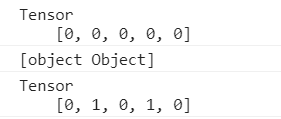# Tensorflow.js 模型

function predict(input) {
// y = a * x ^ 2 + b * x + c
// More on tf.tidy in the next section
return tf.tidy(() => {
const x = tf.scalar(input);

const ax2 = a.mul(x.square());
const bx = b.mul(x);

return y;
});
}

const a = tf.scalar(2);
const b = tf.scalar(4);
const c = tf.scalar(8);

const result = predict(2);
result.print()


const model = tf.sequential();
tf.layers.simpleRNN({
units: 20,
recurrentInitializer: 'GlorotNormal',
inputShape: [80, 4]
})
);

const optimizer = tf.train.sgd(LEARNING_RATE);
model.compile({optimizer, loss: 'categoricalCrossentropy'});
model.fit({x: data, y: labels)});

# Tensorflow.js 内存管理

dispose

const x = tf.tensor2d([[0.0, 2.0], [4.0, 6.0]]);
const x_squared = x.square();

x.dispose();
x_squared.dispose();


tf.tidy

tf.tidy执行一个函数并清除所有创建的中间张量，释放它们的GPU内存。 它不清除内部函数的返回值。

const average = tf.tidy(() => {
const y = tf.tensor1d([1.0, 2.0, 3.0, 4.0]);
const z = tf.ones();

return y.sub(z).square().mean();
});

average.print()


tf.tidy不会清理变量。变量通常持续到机器学习模型的整个生命周期，因此TensorFlow.js不会清理它们，即使它们是在tidy中创建的。不过，您可以手动调用dispose处理它们。

# Tensorflow.js 拟合曲线

## 运行代码

$git clone https://github.com/tensorflow/tfjs-examples$ cd tfjs-examples/polynomial-regression-core
$yarn$ yarn watch


## 输入数据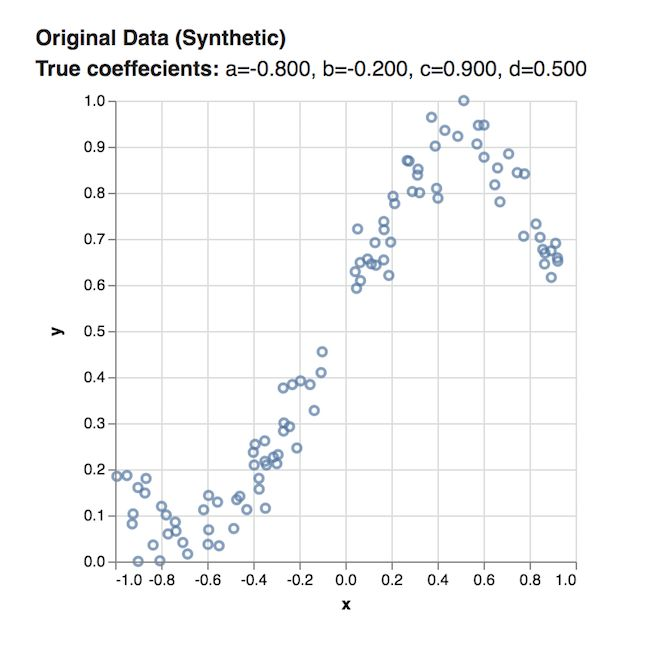## 第1步：设置变量

const a = tf.variable(tf.scalar(Math.random()));
const b = tf.variable(tf.scalar(Math.random()));
const c = tf.variable(tf.scalar(Math.random()));
const d = tf.variable(tf.scalar(Math.random()));


## 第2步：建立模型

function predict(x) {
// y = a * x ^ 3 + b * x ^ 2 + c * x + d
return tf.tidy(() => {
return a.mul(x.pow(tf.scalar(3))) // a * x^3
.add(b.mul(x.square())) // + b * x ^ 2
.add(c.mul(x)) // + c * x
});
}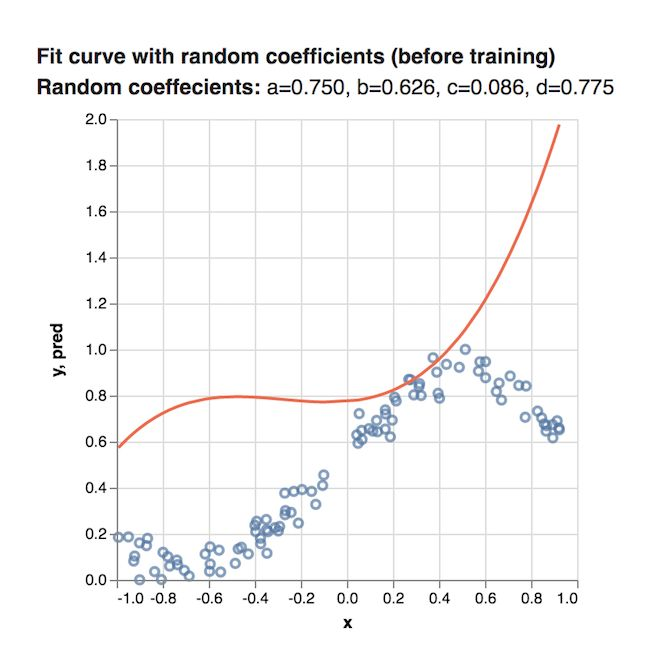## 第3步：训练模型

• 损失函数（loss function）：这个损失函数代表了给定多项式和数据的匹配程度。 损失函数值越小，那么这个多项式和数据就跟匹配。
• 优化器（optimizer）：这个优化器实现了一个算法，它会基于损失函数的输出来修正系数值。所以优化器的目的就是尽可能的减小损失函数的值。
• 训练迭代器（traing loop）：即它会不断地运行这个优化器来减少损失函数。

## 定义损失函数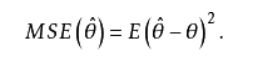function loss(predictions, labels) {
// 将labels（实际的值）进行抽象
// 然后获取平均数.
const meanSquareError = predictions.sub(labels).square().mean();
return meanSquareError;
}

## 定义优化器

TensorFlow.js提供了一个很方便的函数用来实现SGD，所以你不需要担心自己不会这些特别复杂的数学运算。 即 tf.train.sdg 将一个学习率（learning rate）作为输入，然后返回一个SGDOptimizer对象，它与优化损失函数的值是有关的。

const learningRate = 0.5;
const optimizer = tf.train.sgd(learningRate);


## 定义训练循环

function train(xs, ys, numIterations = 75) {

const learningRate = 0.5;
const optimizer = tf.train.sgd(learningRate);

for (let iter = 0; iter < numIterations; iter++) {
optimizer.minimize(() => {
const predsYs = predict(xs);
return loss(predsYs, ys);
});
}


function train(xs, ys, numIterations) {
...
}


const learningRate = 0.5;
const optimizer = tf.train.sgd(learningRate);


for (let iter = 0; iter < numIterations; iter++) {
optimizer.minimize(() => {
const predsYs = predict(xs);
return loss(predsYs, ys);
});
}


minimize 接受了一个函数作为参数，这个函数做了下面的两件事情：

1、首先它对所有的x值通过我们在之前定义的pridict函数预测了y值。

2、然后它通过我们之前定义的损失函数返回了这些预测的均方误差。

minimize函数之后会自动调整这些变量（即系数a、b、c、d）来使得损失函数更小。

## 查看结果！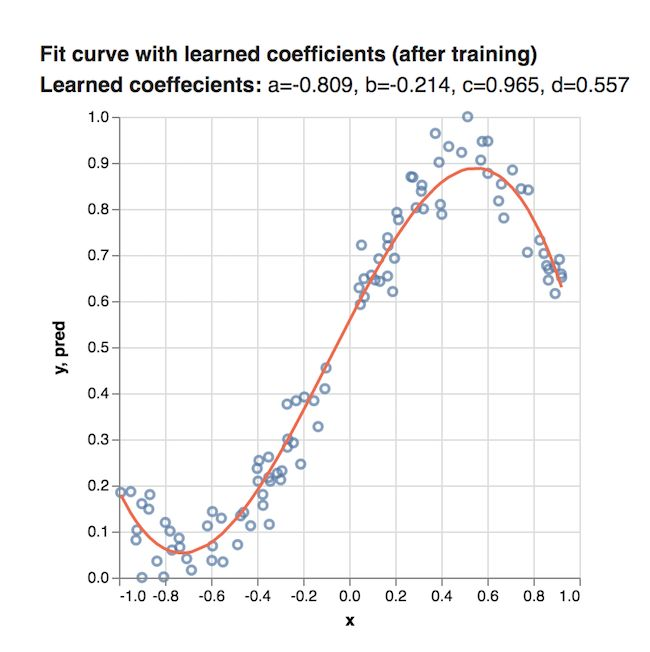# Tensorflow.js 图片训练

## 一、运行代码

$git clone https://github.com/tensorflow/tfjs-examples$ cd tfjs-examples/mnist
$yarn$ yarn watch

## 二、数据相关1、nextTrainBatch(batchSize)： 从训练数据中返回一批任意的图片以及他们的标识。

2、nextTestBatch(batchSize):  从测试数据中返回一批图片以及他们的标识。

## 三、创建模型

首先，我们需要使用tf.sequential先初始化一个sequential模型：

const model = tf.sequential();

## 四、添加第一层

model.add(tf.layers.conv2d({
inputShape: [28, 28, 1],
kernelSize: 5,
filters: 8,
strides: 1,
activation: 'relu',
kernelInitializer: 'VarianceScaling'
}));

• inputShape。这个数据的形状将回流入模型的第一层。在这个示例中，我们的MNIST例子是28 x 28像素的黑白图片，这个关于图片的特定的格式即[row, column, depth]，所以我们想要配置一个[28, 28, 1]的形状，其中28行和28列是这个数字在每个维度上的像素数，且其深度为1，这是因为我们的图片只有1个颜色:
• kernelSize。划过卷积层过滤窗口的数量将会被应用到输入数据中去。这里，我们设置了kernalSize的值为5，也就是指定了一个5 x 5的卷积窗口。
• filters。这个kernelSize的过滤窗口的数量将会被应用到输入数据中，我们这里将8个过滤器应用到数据中。
• strides。 即滑动窗口每一步的步长。比如每当过滤器移动过图片时将会由多少像素的变化。这里，我们指定其步长为1，这意味着每一步都是1像素的移动。
• activation。这个activation函数将会在卷积完成之后被应用到数据上。在这个例子中，我们应用了relu函数，这个函数在机器学习中是一个非常常见的激活函数。
• kernelInitializer。这个方法对于训练动态的模型是非常重要的，他被用于任意地初始化模型的weights。我们这里将不会深入细节来讲，但是 VarianceScaling （即这里用的）真的是一个初始化非常好的选择。

## 五、添加第二层

model.add(tf.layers.maxPooling2d({
poolSize: [2, 2],
strides: [2, 2]
}));
• poolSize。这个滑动池窗口的数量将会被应用到输入的数据中。这里我们设置poolSize为[2, 2]，所以这就意味着池化层将会对输入数据应用2x2的窗口。
• strides。 这个池化层的步长大小。比如，当每次挪开输入数据时窗口需要移动多少像素。这里我们指定strides为[2, 2]，这就意味着过滤器将会以在水平方向和竖直方向上同时移动2个像素的方式来划过图片。

注意：因为poolSize和strides都是2x2，所以池化层空口将会完全不会重叠。这也就意味着池化层将会把激活的大小从上一层减少一半。

## 六、添加剩下的层

model.add(tf.layers.conv2d({
kernelSize: 5,
filters: 16,
strides: 1,
activation: 'relu',
kernelInitializer: 'VarianceScaling'
}));

poolSize: [2, 2],
strides: [2, 2]
}));


model.add(tf.layers.flatten());


model.add(tf.layers.dense({
units: 10,
kernelInitializer: 'VarianceScaling',
activation: 'softmax'
}));


• units. 激活输出的数量。由于这是最后一层，我们正在做10个类别的分类任务（数字0-9），因此我们在这里使用10个units。 （有时units被称为神经元的数量，但我们会避免使用该术语。）
• kernelInitializer. 我们将对dense层使用与卷积层相同的VarianceScaling初始化策略。
• activation. 分类任务的最后一层的激活函数通常是 softmax。 Softmax将我们的10维输出向量归一化为概率分布，使得我们10个类中的每个都有一个概率值。

## 定义优化器

const LEARNING_RATE = 0.15;
const optimizer = tf.train.sgd(LEARNING_RATE);


## 定义损失函数

|class | 0 | 1 | 2 | 3 | 4 | 5 | 6 | 7 | 8 | 9 | |----------|---|---|---|---|---|---|---|---|---|---| |label | 0 | 0 | 0 | 0 | 0 | 0 | 0 | 1 | 0 | 0 | |prediction|.1 |.01|.01|.01|.20|.01|.01|.60|.03|.02|

## 编译模型

model.compile({
optimizer: optimizer,
loss: 'categoricalCrossentropy',
metrics: ['accuracy'],
});


## 配置批量大小

const BATCH_SIZE = 64;
const TRAIN_BATCHES = 100;
const TEST_BATCH_SIZE = 1000;
const TEST_ITERATION_FREQUENCY = 5;


## 编写训练循环

for (let i = 0; i < TRAIN_BATCHES; i++) {
const batch = data.nextTrainBatch(BATCH_SIZE);

let testBatch;
let validationData;

if (i % TEST_ITERATION_FREQUENCY === 0) {
testBatch = data.nextTestBatch(TEST_BATCH_SIZE);
validationData = [
testBatch.xs.reshape([TEST_BATCH_SIZE, 28, 28, 1]), testBatch.labels
];
}

const history = await model.fit(
batch.xs.reshape([BATCH_SIZE, 28, 28, 1]),
batch.labels,
{
batchSize: BATCH_SIZE,
validationData,
epochs: 1
});

const loss = history.history.loss;
const accuracy = history.history.acc;

}


const batch = data.nextTrainBatch(BATCH_SIZE);

• 每5个step（TEST_ITERATION_FREQUENCY），我们构造一次validationData，这是一个包含一批来自MNIST测试集的图像及其相应标签这两个元素的数组，我们将使用这些数据来评估模型的准确性：
if (i % TEST_ITERATION_FREQUENCY === 0) {
testBatch = data.nextTestBatch(TEST_BATCH_SIZE);
validationData = [
testBatch.xs.reshape([TEST_BATCH_SIZE, 28, 28, 1]),
testBatch.labels
];
}


model.fit是模型训练和参数实际更新的地方。

  const history = await model.fit(
batch.xs.reshape([BATCH_SIZE, 28, 28, 1]), batch.labels,
{batchSize: BATCH_SIZE, validationData: validationData, epochs: 1});


• X. 输入图像数据。请记住，我们分批量提供样本，因此我们必须告诉fit函数batch有多大。 MnistData.nextTrainBatch返回形状为[BATCH_SIZE，784]的图像 —— 所有的图像数据是长度为784（28 * 28）的一维向量。但是，我们的模型预期图像数据的形状为[BATCH_SIZE，28,28,1]，因此我们需要使用reshape函数。
• y. 我们的标签;每个图像的正确数字分类。
• BATCHSIZE. 每个训练batch中包含多少个图像。之前我们在这里设置的BATCH_SIZE是64。
• validationData. 每隔TEST_ITERATION_FREQUENCY（这里是5）个Batch，我们构建的验证集。该数据的形状为[TEST_BATCH_SIZE，28,28,1]。之前，我们设置了1000的TEST_BATCH_SIZE。我们的评估度量（准确度）将在此数据集上计算。
• epochs. 批量执行的训练次数。由于我们分批把数据馈送到fit函数，所以我们希望它每次仅从这个batch上进行训练。

const loss = history.history.loss;
const accuracy = history.history.acc;


## 查看结果！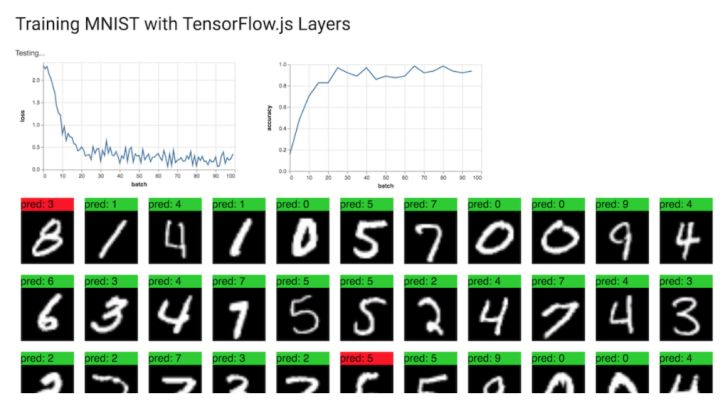# TensorFlow.js 导入Keras模型

Keras模型（通常通过Python API创建）的存储格式可以有多种， 其中“整个模型”（即架构+权重+优化器状态都存在一个文件内）格式可以转换为 TensorFlow.js Layers格式，可以直接加载到TensorFlow.js中进行推理或进一步训练。

TensorFlow.js Layers格式是一个包含model.json文件和一组二进制格式的权重文件分片的目录。 model.json文件包含模型拓扑结构（又名“体系结构”或“图”：层的描述以及它们如何连接）以及权重文件的清单。

pip install tensorflowjs

1、将Keras模型转换为TF.js Layers格式

Keras模型通常使用model.save(filepath)保存，它生成一个包含模型拓扑结构和权重的HDF5（.h5）文件。要将这样的文件转换为TF.js Layer格式，请运行以下命令，其中path / to / my_model.h5是Keras .h5源文件，path / to / tfjs_target_dir是TF.js文件的输出目录：

# bash

tensorflowjs_converter --input_format keras \
path/to/my_model.h5 \
path/to/tfjs_target_dir


# Python

import tensorflowjs as tfjs

def train(...):
model = keras.models.Sequential()   # for example
...
model.compile(...)
model.fit(...)
tfjs.converters.save_keras_model(model, tfjs_target_dir)

2、将模型加载到TensorFlow.js中

// JavaScript

import * as tf from '@tensorflow/tfjs';



// JavaScript

const example = tf.fromPixels(webcamElement);  // for example
const prediction = model.predict(example);


## 支持的功能

TensorFlow.js Layers目前仅支持使用标准Keras构造的Keras模型。使用了不受支持的操作或层（例如自定义层，Lambda层，自定义损失或自定义指标）的模型将无法自动导入，因为它们所依赖的Python代码无法正确地转换为JavaScript。

# 机器学习资源

## 数学概念

2017-12-19 09:32:29 kwame211 阅读数 317
• ###### AI时代，机器学习该如何入门？

机器学习入门视频教程，该课程会告诉大家如何入门机器学习，掌握机器学习必要的基础知识，了解机器学习路径。对于机器学习，很多人的观点是：机器学习技术是今后所有技术人员都绕不过的一个门槛。 那么，普通程序员该学习机器学作为一名对机器学习心有向往的程序员，我该以什么样的姿势开始呢？不妨看看该课程。

7185 人正在学习 去看看 CSDN讲师## Scikit-learnPython已经成为数学、科学和统计方面的首选编程语言，因为它易于采用，而且几乎任何应用程序都可以使用这些库。scikit- learn通过在几个现有的Python包——numpy、SciPy和matplotlib——上构建数学和科学工作，利用了这一广度。生成的库可以用于交互式的“工作台”应用程序，或者嵌入到其他软件中并重新使用。该工具包在BSD许可下可用，因此它是完全开放和可重用的。

Project: scikit-learn

## Shogun(幕府)Project:Shogun

## Accord.Net FrameworkAccord，机器学习和信号处理框架。.Net是之前一个项目的扩展，协议包括一组用于处理音频信号和图像流的库(如视频)。它的视觉处理算法可以用于人脸检测、拼接图像或追踪移动对象等任务。协议还包括提供更传统的机器学习功能的库，从神经网络到决策树系统。

Project:Accord.Net Framework

## Apache MahoutProject:Apache Mahout

## Spark MLlibProject:Spark MLlib

## H2OProject:H2O

## Cloudera OryxProject:Cloudera Oryx

## GoLearnProject:GoLearn

## WekaProject:Weka

## Deeplearn.jsProject:Deeplearn.js

## ConvNetJSProject:ConvNetJS

2017-03-18 22:01:34 u012986684 阅读数 939
• ###### AI时代，机器学习该如何入门？

机器学习入门视频教程，该课程会告诉大家如何入门机器学习，掌握机器学习必要的基础知识，了解机器学习路径。对于机器学习，很多人的观点是：机器学习技术是今后所有技术人员都绕不过的一个门槛。 那么，普通程序员该学习机器学作为一名对机器学习心有向往的程序员，我该以什么样的姿势开始呢？不妨看看该课程。

7185 人正在学习 去看看 CSDN讲师

1、定义：研究开发一系列的算法，不需要外部明显的指示，只需要数据来学习，建模，并且利用建好的模型和新的输入来预测的学科。

2、目前的应用：

3、Demo:

2017-07-07 15:28:07 u011001084 阅读数 310
• ###### AI时代，机器学习该如何入门？

机器学习入门视频教程，该课程会告诉大家如何入门机器学习，掌握机器学习必要的基础知识，了解机器学习路径。对于机器学习，很多人的观点是：机器学习技术是今后所有技术人员都绕不过的一个门槛。 那么，普通程序员该学习机器学作为一名对机器学习心有向往的程序员，我该以什么样的姿势开始呢？不妨看看该课程。

7185 人正在学习 去看看 CSDN讲师

https://mp.weixin.qq.com/s/N6aEUn7J2H48J8XZ4pmvAg

JAVASCRIPT？！我难道不应该用 Python 么？我难道要用 JavaScript 去做如此复杂的运算？难道我不应该使用 Python 或者 R 语言么？scikit-learn 算法库会不会不能在 JavaScript 中使用？简单来说：基于 JavaScript 的机器学习完全没有问题。

为什么在机器学习领域没有提到 JavaScript 呢？
1. JavaScript 很慢。（完全错误的观念 !?! ）

2. JavaScript 很难进行矩阵操作。（但是有很多库的，比如math.js

3. JavaScript 仅仅被认为是用来做 web 开发的。（Node.js 默默的笑了）

4. 机器学习中很多库都是基于 Python 开发的。（那是因为 JavaScript 开发者并没有在场）

1. brain.js（神经网络）

2. Synaptic（神经网络）

3. Natural（自然语言处理）

4. ConvNetJS（卷积神经网络）

5. mljs（一种具有多个函数方法的子库）

开启 JavaScript 的机器学习之路

$yarn add ml-regression csvtojson 或者你更喜欢 npm： $ npm install ml-regression csvtojson

ml-regression所做的事正如它的名字那样，机器学习线性回归库。

csvtojson是在node.js环境中的一个cvs数据解析器，它可以在你加载完cvs 数据后将其快速的转换为JSON

const ml = require('ml-regression');
const csv = require('csvtojson');
const SLR = ml.SLR; // 简单线性回归
const csvFilePath = 'advertising.csv'; // 数据文件
let csvData = [], // 已解析的数据
X = [], // 输入
y = []; // 输出
let regressionModel;

csv()
.fromFile(csvFilePath)
.on('json', (jsonObj) => {
csvData.push(jsonObj);
})
.on('done', () => {
dressData(); // 从 JSON 对象中获取数据点
performRegression();
});

function dressData() {
/**
* 一个数据对象应该这样:
* {
*   TV: "10",
*   Newspaper: "20",
*   "Sales": "1000"
* }
*
* 因此，在添加数据点的同时，
* 我们需要将 String 类型的值解析为 Float 类型。
*/
csvData.forEach((row) => {
y.push(f(row.Sales));
});
}
function f(s) {
return parseFloat(s);
}

function performRegression() {
regressionModel = new SLR(X, y); // 基于训练数据来训练模型
console.log(regressionModel.toString(3));
predictOutput();
}

regressionModel有一个toString方法，它所接收的参数代表输出值浮点数的精度。

predictOutput方法能够接收所输入的值，并且向终端输出所预测的值。

function predictOutput() {
rl.question('Enter input X for prediction (Press CTRL+C to exit) : ', (answer) => {
console.log(At X = ${answer}, y =${regressionModel.predict(parseFloat(answer))});
predictOutput();
});
}

const readline = require('readline'); // 同时预测用户的输入值
input: process.stdin,
output: process.stdout
});

const ml = require('ml-regression');
const csv = require('csvtojson');
const SLR = ml.SLR; // 简单线性回归
const csvFilePath = 'advertising.csv'; // 数据
let csvData = [], // 已解析的数据
X = [], // 输入
y = []; // 输出
let regressionModel;
input: process.stdin,
output: process.stdout
});
csv()
.fromFile(csvFilePath)
.on('json', (jsonObj) => {
csvData.push(jsonObj);
})
.on('done', () => {
dressData(); // 从 JSON 对象中获取数据点
performRegression();
});
function performRegression() {
regressionModel = new SLR(X, y); // 基于训练数据来训练模型
console.log(regressionModel.toString(3));
predictOutput();
}
function dressData() {
/**
* 一个数据对象应该这样：
* {
*   TV: "10",
*   Newspaper: "20",
*   "Sales": "1000"
* }
*
* 因此，在添加数据点的同时，
* 我们需要将 String 类型的值解析为 Float 类型。
*/
csvData.forEach((row) => {
y.push(f(row.Sales));
});
}
function f(s) {
return parseFloat(s);
}
function predictOutput() {
rl.question('Enter input X for prediction (Press CTRL+C to exit) : ', (answer) => {
console.log(At X = ${answer}, y =${regressionModel.predict(parseFloat(answer))});
predictOutput();
});
}

\$ node index.js
f(x) = 0.202 * x + 9.31
Enter input X for prediction (Press CTRL+C to exit) : 151.5
At X = 151.5, y =  39.98974927911285
Enter input X for prediction (Press CTRL+C to exit) :

PS: 我将使用 ml 和其他的库（上面所列出的那些）在各种数据集上执行目前比较流行的机器学习算法。请时刻关注我的动态，获取最新的机器学习教程。

英文原文链接

Machine Learning with JavaScript : Part 1

https://hackernoon.com/machine-learning-with-javascript-part-1-9b97f3ed4fe5